LINCON Statement
LINCON constraint <, constraint ...> ;
where constraint represents one of the following:number operator linear-termlinear-term operator number

and linear-term is

<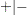><coefficient> parameter <<><coefficient> parameter ...>

The LINCON statement specifies a set of linear equality or inequality constraints of the following form: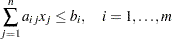The constraints must be separated by commas. Each linear constraintin the statement consists of a linear combination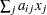of a subset of theparameters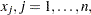and a constant value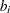separated by a comparison operator. Valid operators are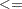, <,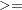, >, andor, equivalently, LE, LT, GE, GT, and EQ. PROC CALIS cannot enforce the strict inequalities < or >. Note that the coefficients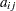in the linear combination must be constant numbers and must be followed by an asterisk and the name of a parameter (that is, listed in the PARMS, main, or subsidiary model specification statements). The following is an example of the LINCON statement that sets a linear constraint on parameters x1 and x2:

```lincon       x1 + 3 * x2 <= 1;
```

Although you can easily express boundary constraints in LINCON statements, for many applications it is much more convenient to specify both the BOUNDS and the LINCON statements in the same PROC CALIS call.